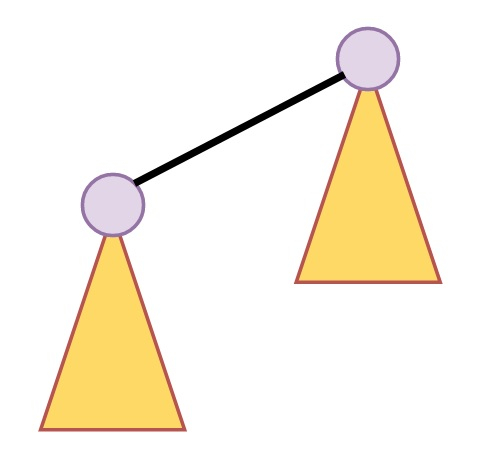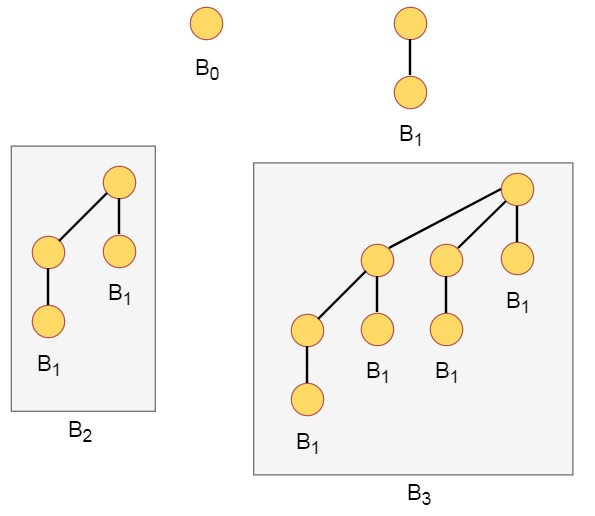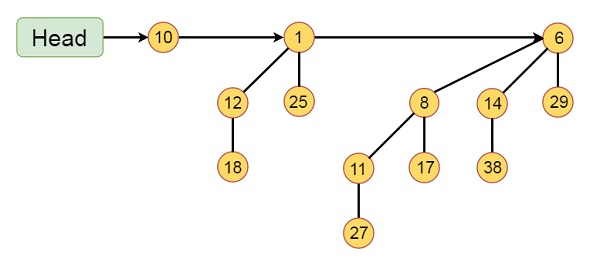# Binomial Heaps in Data Structure

A binomial Heap is a collection of Binomial Trees. A binomial tree Bk is an ordered tree defined recursively. A binomial Tree B0 is consists of a single node.

A binomial tree Bk is consisting of two binomial tree Bk-1. That are linked together. The root of one is the left most child of the root of the otherSome binomial heaps are like below −Some properties of binomial trees are −

• Binomial tree with Bk has 2k nodes.

• Height of the tree is k

• There are exactly $$\left(\begin{array}{c}k\ j\end{array}\right)$$ nodes at depth i for all i in range 0 to k

## Binomial Heap

A binomial heap H is a set of binomial trees. There are some properties.

• Each binomial tree in H is heap-ordered. So the key of a node is greater than or equal to the key of its parent.

• There is at most one binomial tree in H, whose root has a given degree.

## Example of Binomial HeapThis binomial Heap H consists of binomial trees B0, B2 and B3. Which have 1, 4 and 8 nodes respectively. And in total n = 13 nodes. The root of binomial trees are linked by a linked list in order of increasing degree